Levels »

# Lasciate Ogne Speranza, Voi Ch’Intrate

### The Pit, Elevation: -12.8m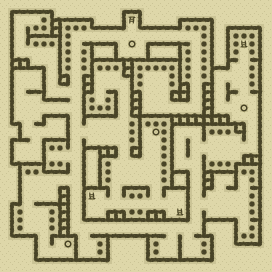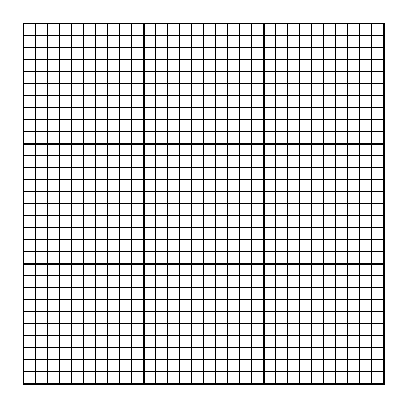### Items

•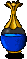2 Clear Blue Potions (18, 8) - (11, 11) (in Red Velvet Bag)
•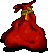Red Velvet Bag (11, 11)

### Monsters

•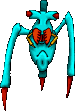38 Skitters (Random rate: 4) (13, 3) - (17, 3) - (23, 4) - (5, 5) - (3, 6) - (4, 6) - (23, 6) - (29, 8) - (2, 9) - (17, 9) - (19, 9) - (26, 9) - (29, 9) - (18, 10) - (11, 11) - (26, 11) - (6, 14) - (12, 14) - (22, 15) - (11, 16) - (18, 16) - (21, 16) - (2, 17) - (21, 17) - (21, 18) - (29, 21) - (29, 22) - (11, 24) - (20, 24) - (2, 25) - (3, 26) - (29, 26) - (29, 27) - (19, 29) - (6, 30) - (6, 30) - (19, 30) - (23, 30)
•4 Sentinels (23, 10) - (6, 21) - (23, 21) - (8, 24)
•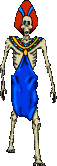15 Ghasts (Random rate: 1) (3, 1) - (29, 5) - (6, 10) - (2, 12) - (12, 15) - (27, 16) - (27, 17) - (27, 18) - (27, 21) - (15, 24) - (4, 25) - (14, 27) - (10, 29) - (23, 29) - (10, 30)

### Locations

• South-facing Sentinel barrier (23, 21)
• East-facing Sentinel barrier (6, 21)
• 2 North-facing Sentinel barriers (23, 10) - (8, 24)
• 4 Save runes(15, 4) - (29, 12) - (18, 15) - (7, 29)